Exact Algorithms for Variants of Satisfiability and Colouring Problems Bjarke Skjernaa November 2004

### Abstract:

This dissertation studies exact algorithms for various hard problems and give an overview of not only results but also the techniques used.

In the first part we focus on Satisfiability and several variants of Satisfiability. We present some historical techniques and results. Our main focus in the first part is on a particular variant of Satisfiability, Exact Satisfiability. Exact Satisfiability is the variant of Satisfiability where a clause is satisfied if it contains exactly one true literal. We present an algorithm solving Exact Satisfiability in time, and an algorithm solving Exact 3-Satisfiability, the variant of Exact Satisfiability where each clause contains at most three literals, in time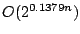. In these algorithms we use a new concept of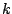-sparse formulas, and we present a new technique called splitting loosely connected formulas, although we do not use the technique in the algorithms.

We present a new program which generates algorithms and corresponding upper bound proofs for variants of Satisfiability, and in particular Exact Satisfiability. The program uses several new techniques which we describe and compare to the techniques used in three other programs generating algorithms and upper bound proofs.

In the second part we focus on another class of NP-complete problems, colouring problems. We present several algorithms for 3-Colouring, and discuss-Colouring in general. We also look at the problem of determining the chromatic number of a graph, which is the minimum number,, such that the graph is-colourable. We present a technique using maximal bipartite subgraphs to solve-Colouring, and prove two bounds on the maximum possible number of maximal bipartite subgraphs of a graph with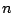vertices: a lower bound of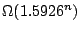and an upper bound of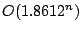. We also present a recent improvement of the upper bound by Byskov and Eppstein, and show a number of applications of this in colouring problems.

In the last part of this dissertation we look at a class of problems unrelated to the above, Graph Distinguishability problems. DIST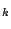is the problem of determining if a graph can be coloured withcolours, such that no non-trivial automorphism of the graph preserves the colouring. We present some results on determining the distinguishing number of a graph, which is the minimum, such that the graph is in DIST. We finish by proving a new result which shows that DISTcan be reduced to DIST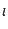for various values ofand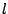Available as PostScript, PDF.